Translation
Reflection
Rotation
Dilation
Composite Transformation
Reflection and Rotation Symmetry
100

Define Translation

When you slide a figure or point.

100

Define Reflection.

When you flip or make a mirror image of a figure or point.

100

Define Rotation

When you turn a figure or point. (can be clockwise or counterclockwise)

100

Define Dilation.

When you enlarge or shrink a figure or point by multiplying it a scale factor.

100

Define Composite Transformation.

When you use more than one transformation on a figure or point.

100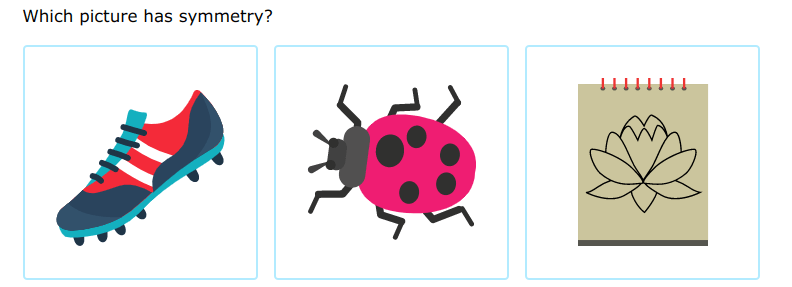Drawing of the flower (3rd image)

200

Describe the translation:

(x-5, y+3)

Slide the figure or point 5 units to the left and 3 units up.

200

What is the algebraic rule for reflecting over the line y=-x.

(x,y) --> (-y, -x)

200

What is the algebraic rule for rotating a figure 90 degrees counterclockwise?

(x,y) ---> (-y, x)

200

What is the algebraic rule when dilating a point with the scale factor 4.

(x,y)---> 4(x,y) ---> (4x, 4y)

200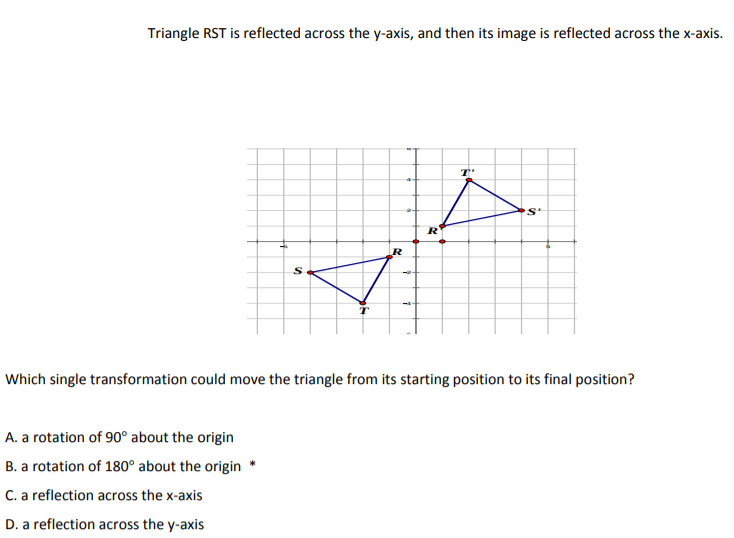B. rotation of 180 about the origin

200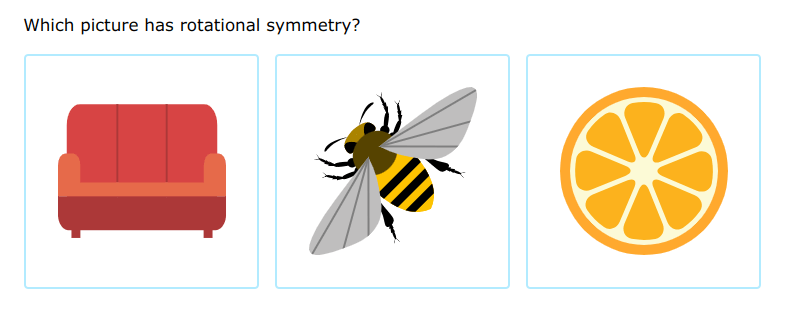Orange (3rd image)

300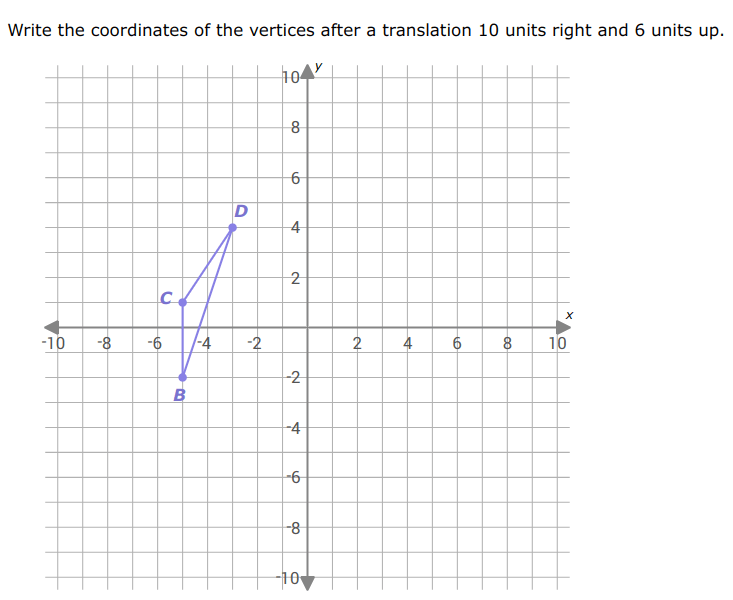B' (5,4)

C' (5,7)

D' (7,10)

300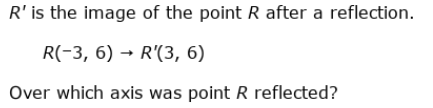Reflection over y-axis

300

What is the algebraic rule for 180 degrees clockwise?

(x,y)----> (-x,-y)

300

Triangle ABC is being dilated by scale factor 1/4. Is the triangle enlarging or shrinking? How do you know?

Shrinking. The scale factor is less than 1, so that is why the triangle is shrinking.

300

What would the algebraic rule be if a figure reflected over the x-axis and rotated 180 degrees?

(x,y) ----> (x,-y) ----> (-x,--y)----> (-x,y)

300

Explain how you know if something has reflection symmetry.

One half of the figure is the reflection of the other half.

400

Complete the table

Write the coordinates of the vertices after a translation 8 units right and 1 unit down.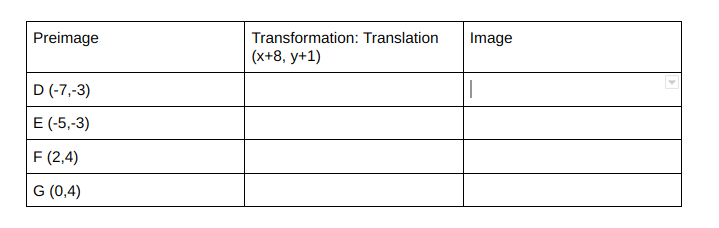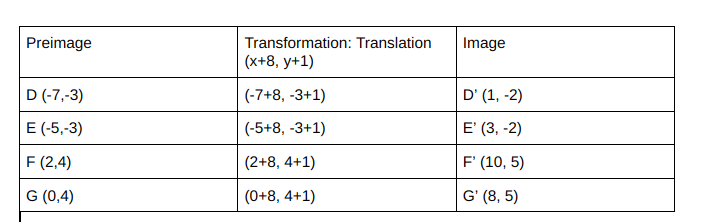400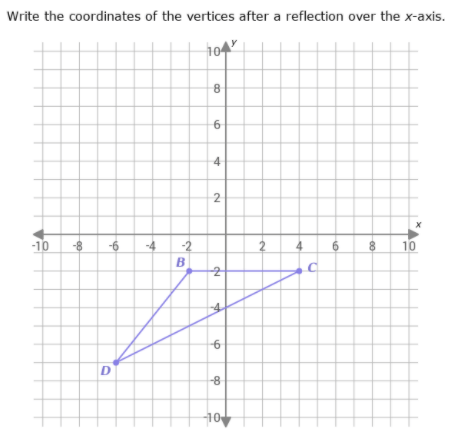B' (-2,2)

C' (4,2)

D' (-6, 7)

400

Point A (-2,3) is the preimage of A'(-3,-2)

What rotation rule happened to go from Point A to Point A' clockwise?

270 clockwise

400

Describe the dilation

(3,6) ----> (6, 12)

Dilated by scale factor 2

400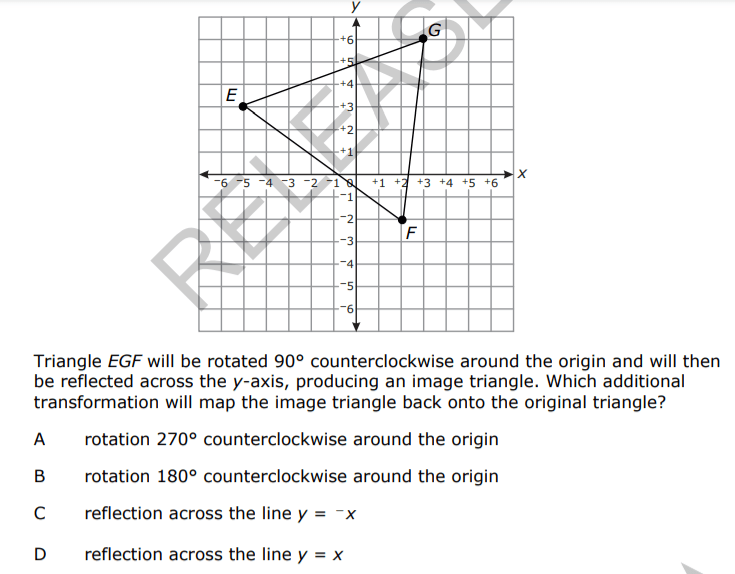D. Reflection across the line y=x

400

Explain how you know a figure has rotation symmetry

The figure looks the same after some rotation.

500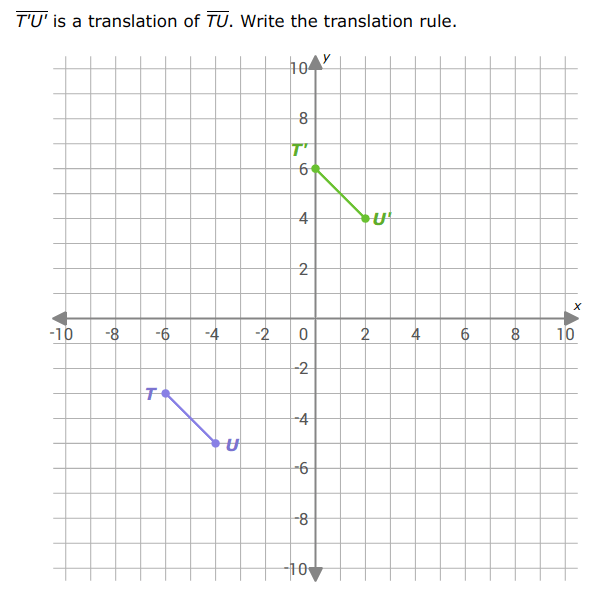(x+6, y+9)

6 units to the right; 9 units up

500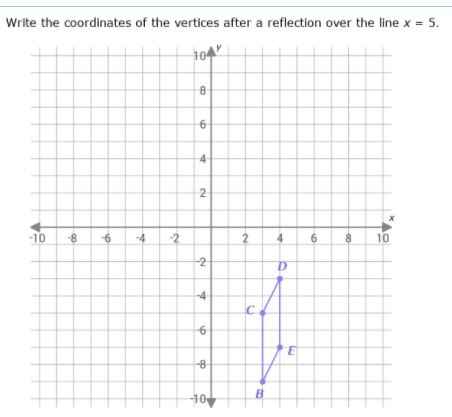B' (7, -9)

C' (7, -5)

D' (6, -3)

E' (6, -7)

500

Describe the rotation.

(2,3) ----> (-2, -3)

180 rotation

500

Point A (10, 15)

Point A' (2, 3)

What is the scale factor?

Dilated by scale factor 1/5

500

A point is reflected over the y-axis then over the x-axis. What is a single transformation that can represent this composite transformation?

180 rotation

500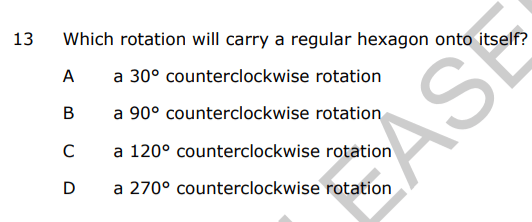C. 120 degrees ccw rotation

Click to zoom